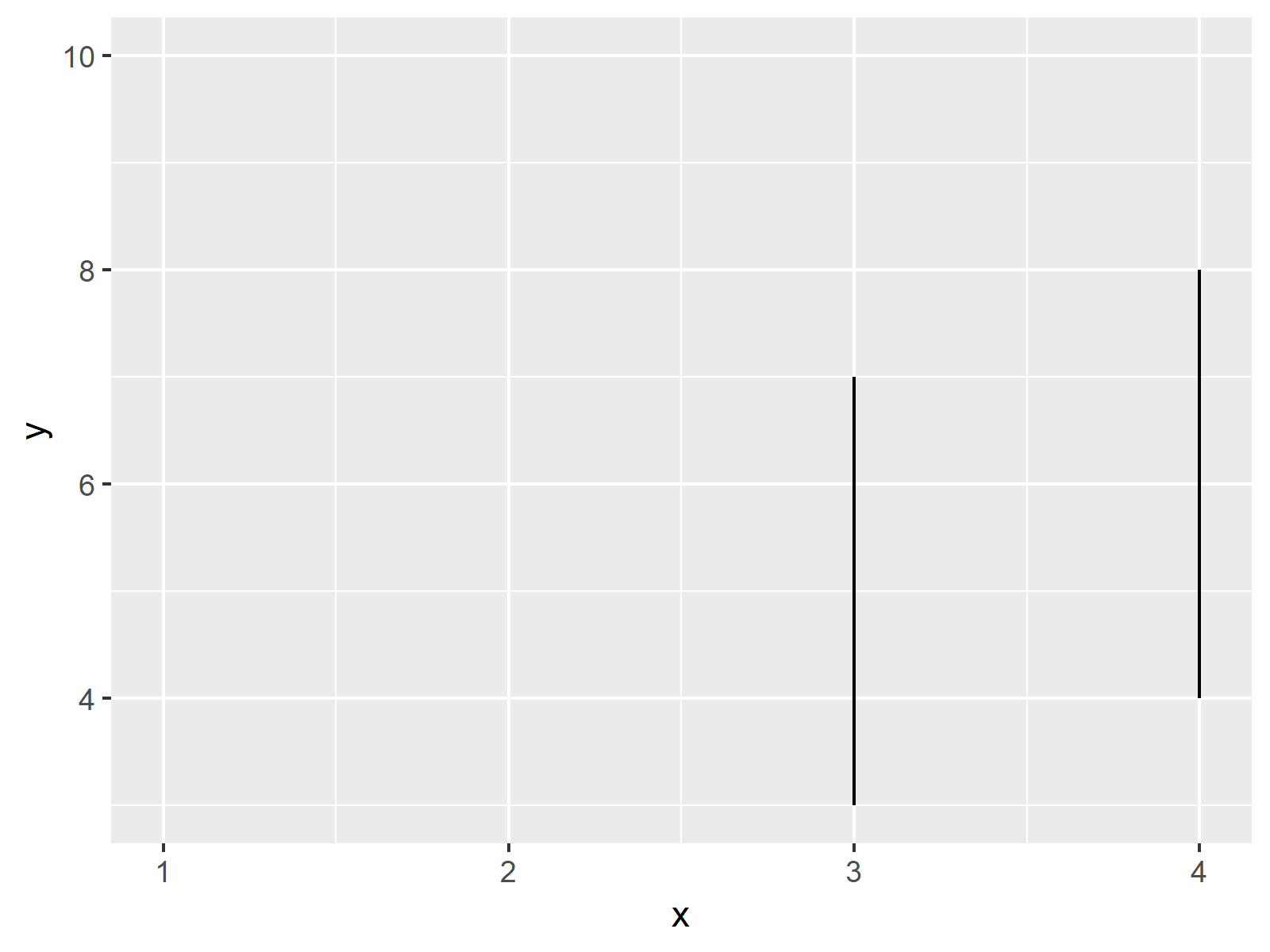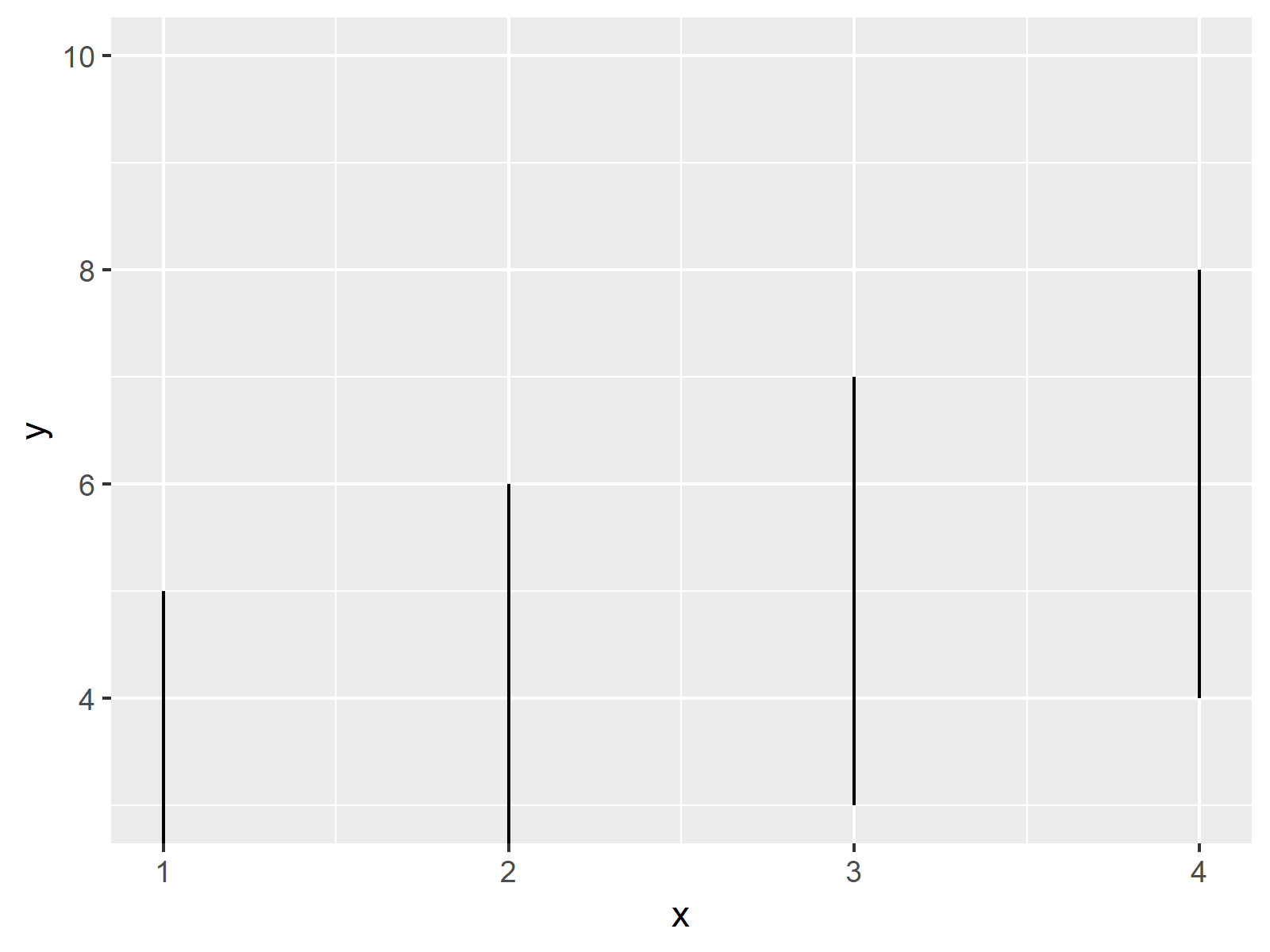# Zoom into ggplot2 Plot without Removing Data in R (Example)

In this R tutorial you’ll learn how to set axis limits of a ggplot2 plot without removing data points.

The post looks as follows:

Here’s the step-by-step process…

## Example Data, Add-On Packages & Basic Plot

As a first step, let’s define some example data:

```data <- data.frame(x = 1:4,                        # Create example data
y = 1:8,
group = rep(letters[1:4], 2))
data                                               # Print example data
#   x y group
# 1 1 1     a
# 2 2 2     b
# 3 3 3     c
# 4 4 4     d
# 5 1 5     a
# 6 2 6     b
# 7 3 7     c
# 8 4 8     d```

The previous output of the RStudio console shows that our example data has three columns. The variables x and y are numeric and the variable group defines the groups of our data points.

If we want to create a graphic of the data with the ggplot2 package, we also have to install and load ggplot2:

```install.packages("ggplot2")                        # Install ggplot2 package

Now, we can plot our data as shown below:

```ggp <- ggplot(data, aes(x, y, group = group)) +    # Create ggplot2 plot without zoom
geom_line()
ggp                                                # Draw plot```The output of the previous R programming syntax is shown in Figure 1 – A basic ggplot2 line plot without any zoom.

Let’s try to zoom in with the next part of the code:

```ggp +                                              # Zoom in & cut off values
ylim(3, 10)
# Warning:
# Removed 2 rows containing missing values (geom_path).```The RStudio console returns a warning message: Removed 2 rows containing missing values (geom_path). Furthermore, some lines of our data are removed entirely from our second graph, even though they should be shown in the zoomed plot…

## Example: Zoom In without Cutting Off Data

This Example illustrates how to zoom in properly, without losing any data points. For this task, we have to use the coord_cartesian argument. Within the coord_cartesian argument, we are defining the axis limits of our ggplot2 plot:

```ggp +                                              # Zoom without cutting values
coord_cartesian(ylim = c(3, 10))```As you can see based on our third plot, this time we haven’t lost any lines of our data, i.e. the first and the second line are still shown.

## Video, Further Resources & Summary

Do you need further explanations on the R programming syntax of this article? Then you could watch the following video of the Statistics Globe YouTube channel. I’m explaining the content of this page in the video:

Please accept YouTube cookies to play this video. By accepting you will be accessing content from YouTube, a service provided by an external third party.If you accept this notice, your choice will be saved and the page will refresh.

In addition, you could read the other R programming tutorials on this homepage. I have published numerous articles already:

In this tutorial, I explained how to zoom into the area of a plot without deleting data in R.

In the present tutorial, we have used a line plot to show how to avoid that the rescaling of a ggplot2 axis causes data to disappear. However, the same logic could be applied to other types of plots such as barplots, boxplots, histograms, and so on.